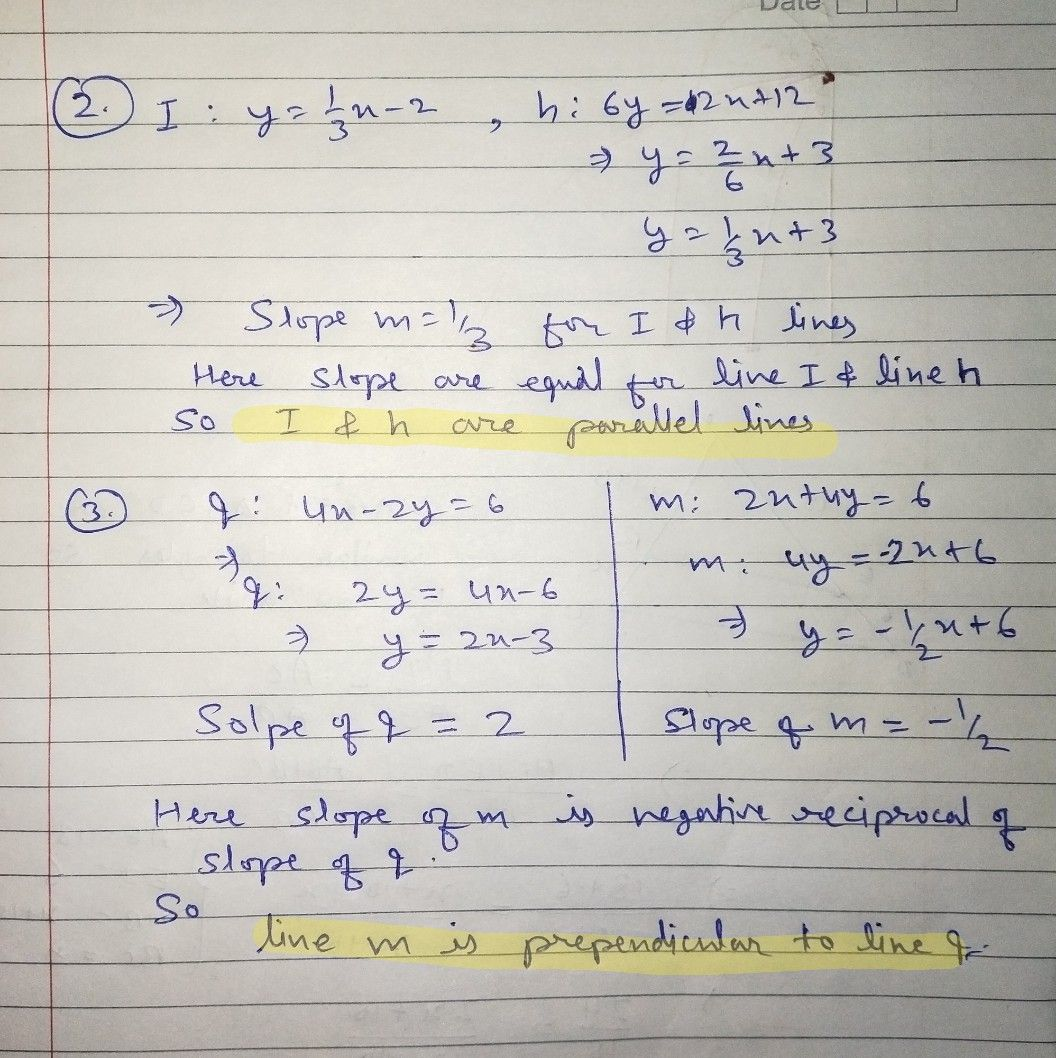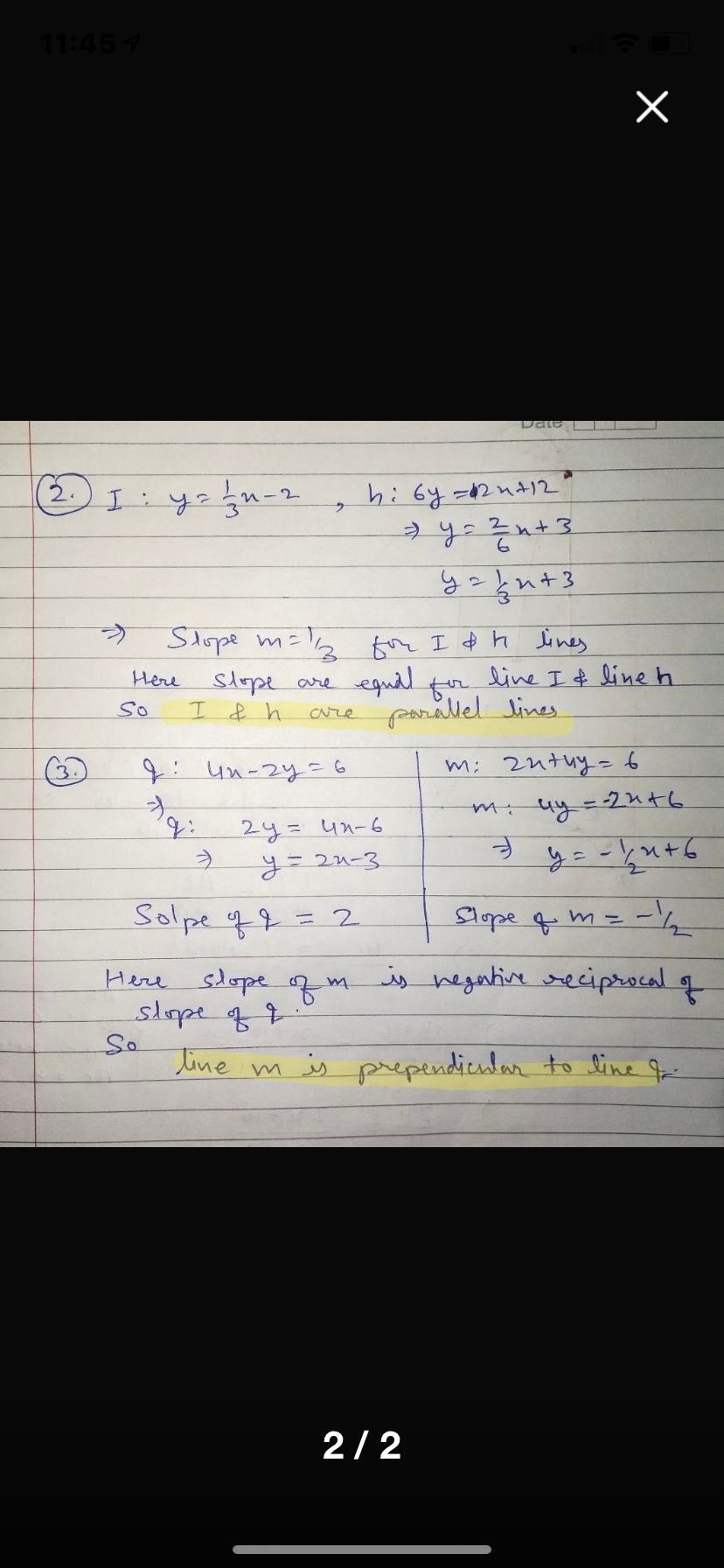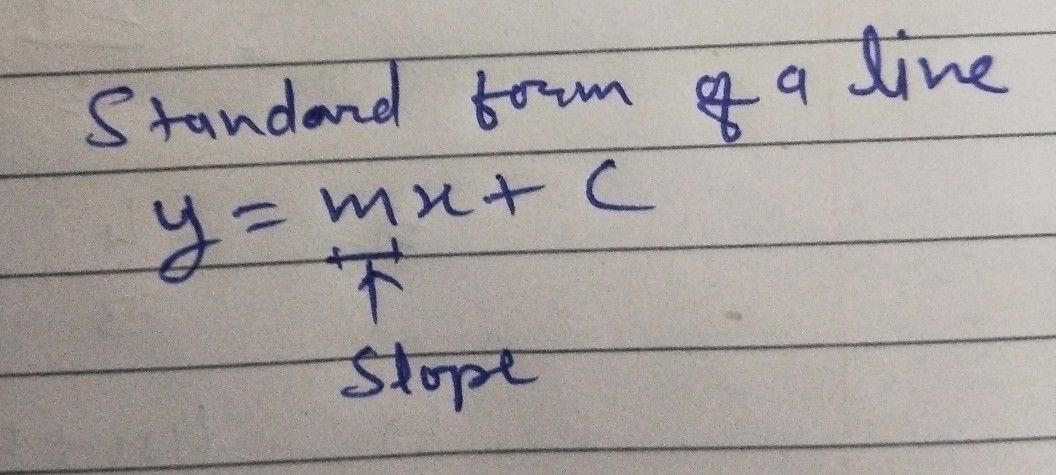Symbol
ProblemAre these equations parallel, perpendicular, or neither? $2$ $ly=$ $y=\dfrac {1} {3}x-2$ $b$ $6y=2x+12$ $3$ $q$ $4x-2y=6$ $m$ $2x+4y=6$
10th-13th grade
Geometry
SolutionQanda teacher - SHIVRAJCheck it dear ?
If it's helpful then evaluate the answer dear and give me a feedback and 5star please dear ?
Your feedback is inspiration for me so please ?StudentThank you so muchQanda teacher - SHIVRAJ
Welcome dear ?Student
What is the slope for number 3?Qanda teacher - SHIVRAJ
Slope of line q is 2 and slope of line m is - 1/2Student
Thank youQanda teacher - SHIVRAJPlease evaluate the answer dear and give me a feedback and 5star please dear
Your feedback is inspiration for me so please ?Student
I will how do I give you a 5 star?